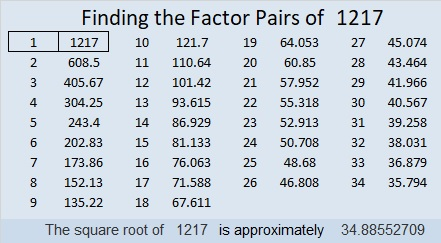# 1217 Squeegee Mystery

The murder weapon for today’s mystery appears to be a squeegee! Study all the clues in the puzzle and see if you can find all the needed factors to solve this mystery. As in every whodunnit, there is only one true solution.Print the puzzles or type the solution in this excel file: 12 factors 1211-1220

Now here are a few facts about the number 1217:

• 1217 is a prime number.
• Prime factorization: 1217 is prime.
• The exponent of prime number 1217 is 1. Adding 1 to that exponent we get (1 + 1) = 2. Therefore 1217 has exactly 2 factors.
• Factors of 1217: 1, 1217
• Factor pairs: 1217 = 1 × 1217
• 1217 has no square factors that allow its square root to be simplified. √1217 ≈ 34.88553

How do we know that 1217 is a prime number? If 1217 were not a prime number, then it would be divisible by at least one prime number less than or equal to √1217 ≈ 34.9. Since 1217 cannot be divided evenly by 2, 3, 5, 7, 11, 13, 17, 19, 23, 29 or 31, we know that 1217 is a prime number.31² + 16² = 1217

1217 is the hypotenuse of a Pythagorean triple:
705-992-1217 calculated from 31² – 16², 2(31)(16), 31² + 16²

Here’s another way we know that 1217 is a prime number: Since its last two digits divided by 4 leave a remainder of 1, and 31² + 16² = 1217 with 31 and 16 having no common prime factors, 1217 will be prime unless it is divisible by a prime number Pythagorean triple hypotenuse less than or equal to √1217 ≈ 34.9. Since 1217 is not divisible by 5, 13, 17, or 29, we know that 1217 is a prime number.

This site uses Akismet to reduce spam. Learn how your comment data is processed.